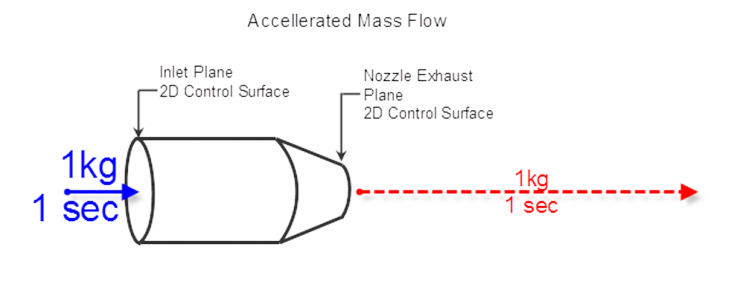Turbojets develop thrust by accelerating mass. They do this by compressing intake air, mixing it with fuel, combusting the mixture and expelling it in a highly accelerated and controlled manner. The result of this is thrust, and its opposite reaction moves the aircraft. The accelerated fluid (combusted air) is quantified as mass flow.

Mass Flow

Mass flow is the measure of the mass of a fluid that passes over a control surface, in a specific frame of time.

Consider: A surface has only 2 dimensions, which constitute an area. The third dimension needed to calculate a volume (and then the mass thereof) is represented by the velocity at which the fluid (air) flows past that surface. In our case, that rate is stated as meters per second (m/s).  If we know the area of the inlet, the density of the fluid, and the velocity that it is travelling at, then we can calculate the mass flowing past that surface in one second.Mass flow is often noted in fluids analysis and engine design as $\dot{m}$, or in text as mdot, and mass dot.

This is equivalent to the following:

$\rho \times A \times V = \dot{m}$.

Where:

$\rho$ [kg/m^3] – the static density of the fluid

A [m^2] – control surface area

V [m/s] – velocity of the fluid.

$\dot{m}$ is then related as mass/time, here as kg/s

Control Surfaces

Control surfaces are simply a fixed imaginary 2D surfaces that the engineer is using to establish the controls for his/her flow calculations. Literally, a frame of reference that can be established (and re-established) in space. In our study of “the Damn Turbofan”, the initial control surface is located at station 1, the leading edge of the inlet. Knowing the 2D area of the inlet allows me to determine the total quantity of the air entering the engine.

An example of this:

$\rho \times A \times V = \dot{m}$.

$1.225 [kg/m^3] \times 0.011 [m^2] \times 350 [m/s] = 5 [kg/s]$

Considerations

One important thing to consider is that like energy, mass cannot be created or destroyed. Therefore, the mass entering the engine is the same as the mass leaving the engine (neglecting the added fuel for the scope of this discussion). The mass can’t exit the engine at a faster or slower rate in a static environment. You may accelerate the flow of molecules by reducing the exit control surface area or really pissing the molecules off by adding energy, but the flow rate of mass exiting remains the same as the flow rate of mass entering.

$\dot{m}9=\dot{m}1$

This is the fundamental principle throughout this study.

The other thing to consider is that somewhere in the engine is a point that is the most restrictive to the fluid. No matter why it is so restrictive, no other surface will permit more mass to pass through any faster. This is the entire problem with choking, which we will discuss later.